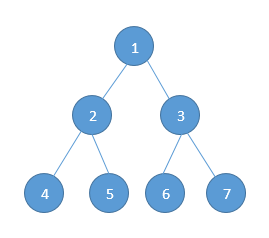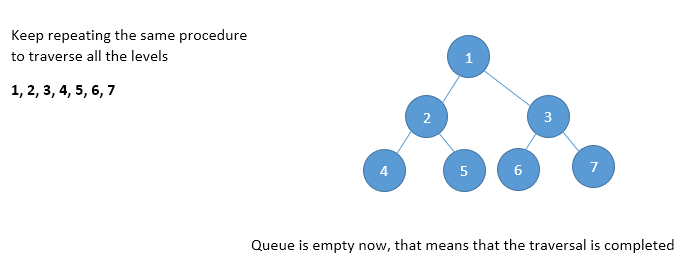Home Theory Level order Traversal in a Binary Tree

# Level order Traversal in a Binary Tree

0 comment

We discussed the basic tree traversals in this post –

We learned about the pre-order traversal, in-order traversal and post-order traversal. These are standard traversals that are related with root, left sub-tree and the right sub-tree. Level order traversal follows an entirely different approach and is not related with the left sub-tree and the right sub-tree. It does not hold the same property for all the nodes and hence recursive technique cannot be applied to level order traversal.

Let us try to understand with the help of our sample tree.In this example, we have 3 levels. So the according to level order traversal –

• Root – 1
• Level 1 – 2, 3
• Level 2 – 4, 5, 6, 7

Finally, we now have the level order traversal as:- 1, 2, 3, 4, 5, 6, 7. The algorithm that we will use is by taking the help of a queue.

• Visit the root
• While traversing level 1, keep all the elements at level l+1 in queue.
• Go to the next level and visit all the nodes at that level
• Repeat this process until all levels are completed.

This can be represented as:-void levelOrder(struct binaryTreeNode * root)
{
// Create a temporary binary tree node
struct binaryTreeNode * temp = NULL;

// Create a queue
struct queue * Q = NULL;

// If the tree is empty we simply need to return
if(root == NULL)
return;

// If the tree is not empty
// We add the root node to the queue
Q = enQueue(Q, root);

// We need to loop until our queue is not empty
while(!isQueueEmpty(Q))
{
// Store the front element of queue in temporary variable
temp = Q -> front -> data;

// Dequeue
Q = deQueue(Q);

// Process the current node
printf("%d ",temp -> data);

// If the left child exists
if(temp -> left)
Q = enQueue(Q, temp -> left);

// If the right child exists, enqueue that also
if(temp -> right)
Q = enQueue(Q, temp -> right);
}

// Delete the queue
free(Q);
}


Time Complexity:- O(n)
Space Complexity:- O(n), Since in the worst case, all the nodes on the entire last level could be in the queue simultaneously.